# WO2020097944A1 - Real power curve-based method for discriminating and compensating yaw error inherent deviation of wind turbine generator set - Google Patents

Real power curve-based method for discriminating and compensating yaw error inherent deviation of wind turbine generator set Download PDF

## Info

Publication number
WO2020097944A1
WO2020097944A1 PCT/CN2018/116072 CN2018116072W WO2020097944A1 WO 2020097944 A1 WO2020097944 A1 WO 2020097944A1 CN 2018116072 W CN2018116072 W CN 2018116072W WO 2020097944 A1 WO2020097944 A1 WO 2020097944A1
Authority
WO
WIPO (PCT)
Prior art keywords
yaw error
interval
data
inherent deviation
wind speed
Prior art date
Application number
PCT/CN2018/116072
Other languages
French (fr)
Chinese (zh)
Inventor

Original Assignee

Priority date (The priority date is an assumption and is not a legal conclusion. Google has not performed a legal analysis and makes no representation as to the accuracy of the date listed.)
Filing date
Publication date
Application filed by 浙江大学 filed Critical 浙江大学
Priority to PCT/CN2018/116072 priority Critical patent/WO2020097944A1/en
Publication of WO2020097944A1 publication Critical patent/WO2020097944A1/en

• 238000000034 methods Methods 0.000 claims abstract description 21
• 238000004458 analytical method Methods 0.000 claims description 126
• 230000000875 corresponding Effects 0.000 claims description 30
• 238000004364 calculation method Methods 0.000 claims description 21
• 238000004422 calculation algorithm Methods 0.000 claims description 16
• 230000001186 cumulative Effects 0.000 claims description 3
• 238000005315 distribution function Methods 0.000 claims description 3
• 238000005457 optimization Methods 0.000 claims description 3
• 230000000153 supplemental Effects 0.000 claims description 3
• 238000001514 detection method Methods 0.000 claims 9
• 238000005259 measurement Methods 0.000 claims 1
• 238000007781 pre-processing Methods 0.000 abstract 1
• 238000004445 quantitative analysis Methods 0.000 abstract 1
• 239000003138 indicators Substances 0.000 description 9
• 238000004088 simulation Methods 0.000 description 7
• 238000007405 data analysis Methods 0.000 description 4
• 238000010248 power generation Methods 0.000 description 4
• 241000873224 Capparaceae Species 0.000 description 3
• 238000010586 diagrams Methods 0.000 description 3
• 238000005516 engineering processes Methods 0.000 description 3
• 239000012080 ambient air Substances 0.000 description 2
• 238000010276 construction Methods 0.000 description 2
• 230000000694 effects Effects 0.000 description 2
• 238000009434 installation Methods 0.000 description 2
• 238000009825 accumulation Methods 0.000 description 1
• 230000015556 catabolic process Effects 0.000 description 1
• 230000001276 controlling effects Effects 0.000 description 1
• 230000004059 degradation Effects 0.000 description 1
• 238000006731 degradation reactions Methods 0.000 description 1
• 238000009472 formulation Methods 0.000 description 1
• 238000007689 inspection Methods 0.000 description 1
• 238000000691 measurement method Methods 0.000 description 1
• 239000000203 mixtures Substances 0.000 description 1
• 238000005070 sampling Methods 0.000 description 1
• 230000000007 visual effect Effects 0.000 description 1

## Images

•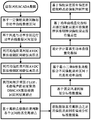•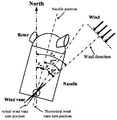•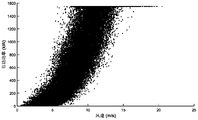•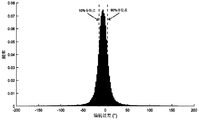•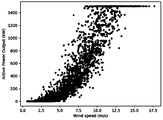•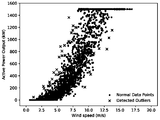•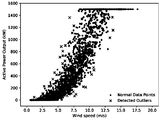•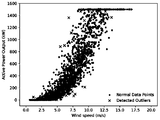••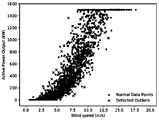•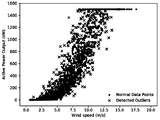••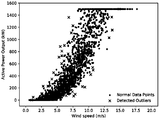•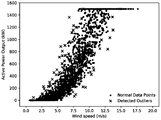•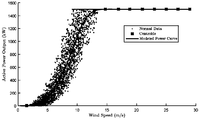•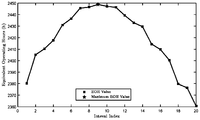•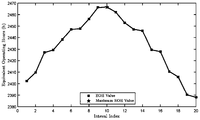## Classifications

• FMECHANICAL ENGINEERING; LIGHTING; HEATING; WEAPONS; BLASTING
• F03MACHINES OR ENGINES FOR LIQUIDS; WIND, SPRING, OR WEIGHT MOTORS; PRODUCING MECHANICAL POWER OR A REACTIVE PROPULSIVE THRUST, NOT OTHERWISE PROVIDED FOR
• F03DWIND MOTORS
• F03D17/00Monitoring or testing of wind motors, e.g. diagnostics
• FMECHANICAL ENGINEERING; LIGHTING; HEATING; WEAPONS; BLASTING
• F03MACHINES OR ENGINES FOR LIQUIDS; WIND, SPRING, OR WEIGHT MOTORS; PRODUCING MECHANICAL POWER OR A REACTIVE PROPULSIVE THRUST, NOT OTHERWISE PROVIDED FOR
• F03DWIND MOTORS
• F03D7/00Controlling wind motors
• F03D7/02Controlling wind motors the wind motors having rotation axis substantially parallel to the air flow entering the rotor
• F03D7/04Automatic control; Regulation
• YGENERAL TAGGING OF NEW TECHNOLOGICAL DEVELOPMENTS; GENERAL TAGGING OF CROSS-SECTIONAL TECHNOLOGIES SPANNING OVER SEVERAL SECTIONS OF THE IPC; TECHNICAL SUBJECTS COVERED BY FORMER USPC CROSS-REFERENCE ART COLLECTIONS [XRACs] AND DIGESTS
• Y02TECHNOLOGIES OR APPLICATIONS FOR MITIGATION OR ADAPTATION AGAINST CLIMATE CHANGE
• Y02EREDUCTION OF GREENHOUSE GAS [GHG] EMISSIONS, RELATED TO ENERGY GENERATION, TRANSMISSION OR DISTRIBUTION
• Y02E10/00Energy generation through renewable energy sources
• Y02E10/70Wind energy
• Y02E10/72Wind turbines with rotation axis in wind direction

## Abstract

A real power curve-based method for discriminating and compensating a yaw error inherent deviation of a wind turbine generator set. Said method comprises: on the basis of real-time operation data, including wind speed, active power, yaw error, etc., of a supervisory control and data acquisition (SCADA) system of a wind turbine generator set, first performing a certain degree of preprocessing on the data and then dividing power curve data according to a certain yaw error interval; in combination with an outlier discrimination method, fitting power curves of different yaw error intervals by means of a real power curve fitting process; further performing a quantitative analysis on different power curves and determining, on the basis of a section determination criterion, the section range of an yaw error inherent deviation value; and finally, compensating the discriminated inherent deviation value to a yaw error measurement value. Said method is based on data driving, and has no special requirements on the operation data of the wind turbine generator set, having strong universality and having a strong application value for improving the performance of the wind turbine generator set.

## Description

(1)装机工人往往不会借助测量设备，而是仅凭经验或目测的方式进行风向仪零刻线位置的校准；(1) The installation workers often do not rely on measuring equipment, but only calibrate the position of the zero line of the wind vane based on experience or visual inspection;
(2)风向仪在实际运行下的反复转动过程中同样可能由于机械原因出现回程误差。这两方面的逐渐积累往往会给偏航误差角度的测量带来较大的误差，从而影响偏航系统的性能。(2) During the repeated rotation of the wind vane in actual operation, the return error may also occur due to mechanical reasons. The gradual accumulation of these two aspects will often bring a large error to the measurement of the yaw error angle, thereby affecting the performance of the yaw system.

1)根据待分析风电机组偏航误差固有偏差辨识及补偿需求，读取相应需求周期内总计N条待分析风电机组的SCADA系统中测量得到的风电机组运行数据信息，该信息包含风速{v i}、有功功率{P i}以及偏航误差{θ i}，将信息数据集记为风电机组偏航误差固有偏差分析数据集{X i}，其中i＝1，2，3，…，N； 1) According to be analyzed wind turbine yaw error inherent bias identification and compensation needs to read the wind turbine operating data within the corresponding demand cycles total SCADA system the N analysis wind turbine to be measured obtained, the information containing Winds {v i }, Active power {P i } and yaw error {θ i }, record the information data set as the wind turbine yaw error inherent deviation analysis data set {X i }, where i = 1, 2, 3, ..., N ;
2)将步骤1)中的风电机组偏航误差固有偏差分析数据集{X i}以一定的偏航误差间隔划分为M个区间，记第k个偏航误差划分区间中的数据个数为N k、偏航误差固有偏差分析数据集为 其中k＝1，2，3，…，M，l＝1，2，3，…，N k2) Divide the yaw error inherent deviation analysis data set {X i } in step 1) into M intervals at a certain yaw error interval, and record the number of data in the kth yaw error division interval as N k , the yaw error inherent deviation analysis data set is Where k = 1, 2, 3, ..., M, l = 1, 2, 3, ..., N k ;
3)基于步骤2)中M个区间的偏航误差固有偏差分析数据集 分别拟合出M条真实功率曲线，记第k个偏航误差区间下的真实功率曲线为{PC k}，其中k＝1，2，3，…，M； 3) Analysis data set based on the inherent deviation of yaw error in M intervals in step 2) Fit the M real power curves separately, and record the real power curve under the k-th yaw error interval as {PC k }, where k = 1, 2, 3, ..., M;
4)分别计算步骤3)中M个偏航误差区间下的真实功率曲线{PC k}各自的量化性能指标PI k，其中k＝1，2，3，…，M； 4) Calculate the respective quantitative performance index PI k of the true power curve {PC k } under M yaw error intervals in step 3), where k = 1, 2, 3, ..., M;
5)通过偏航误差固有偏差辨识准则确定该风电机组的偏航误差固有偏差值θ im的辨识结果，并将该偏差值θ im直接以增量形式补偿到偏航误差实际测量值θ上，得到最终补偿后的偏航误差真实值θ′，即θ′＝θ+θ im5) Determine the identification result of the yaw error inherent deviation value θ im of the wind turbine by the yaw error inherent deviation identification criterion, and directly compensate the deviation value θ im to the actual yaw error measurement value θ in incremental form, The true value of yaw error θ ′ after the final compensation is obtained, that is, θ ′ = θ + θ im ;

2-a)绘制偏航误差{θ i}的频率分布直方图，并基于频率分布直方图的分布情况设定待分析偏航误差范围的下界θ lb和上界θ ub2-a) Plot the frequency distribution histogram of yaw error {θ i }, and set the lower bound θ lb and the upper bound θ ub of the yaw error range to be analyzed based on the distribution of the frequency distribution histogram;
2-b)设定偏航误差固有偏差分析数据集{X i}的区间划分个数M； 2-b) Set the interval division number M of the yaw error inherent deviation analysis data set {X i };
2-c)以 为偏航误差区间划分间隔，对偏航误差固有偏差分析数据集{X i}进行划分，并仅保留风速和功率信息作为偏航误差固有偏差分析数据集 2-c) to To divide the interval for the yaw error interval, divide the yaw error inherent deviation analysis data set {X i }, and keep only the wind speed and power information as the yaw error inherent deviation analysis data set

3-a)设定真实功率曲线获取初始区间k＝1；3-a) Set the real power curve to obtain the initial interval k = 1;
3-b)将第k个偏航误差区间下偏航误差固有偏差分析数据集 中的风速信息和功率信息分别进行最大值-最小值归一化处理，记归一化偏航误差固有偏差分析数据集为 其中l＝1，2，3，…，N k3-b) Analyze the data set of the inherent deviation of yaw error in the k-th yaw error interval The wind speed information and power information in are respectively normalized by the maximum-minimum value, and the normalized yaw error inherent deviation analysis data set is Where l = 1, 2, 3, ..., N k ;
3-c)分别按照一定的风速间隔(ws)和功率间隔(ap)将步骤3-b)中的归一化偏航误差固有偏差分析数据集 划分为M′个区间，并分别记第j个区间中的数据个数为M′ k，seg，j、归一化偏航误差固有偏差分析数据集为 其中seg＝{ws，ap}，j＝1，2，3，…，M′，n＝1，2，3，…，M′ k，seg，j3-c) Analyze the data set of the normalized yaw error inherent deviation in step 3-b) according to a certain wind speed interval (ws) and power interval (ap) respectively Divide into M ′ intervals, and record the number of data in the j-th interval as M ′ k, seg, j , and the normalized yaw error inherent deviation analysis data set as Where seg = {ws, ap}, j = 1, 2, 3, ..., M ', n = 1, 2, 3, ..., M' k, seg, j ;
3-d)利用均值距离判别(AVDC)离群点检测算法分别对步骤3-c)中的2M′个区间 进行疑似离群点检测，并分别记第j个区间的疑似离群点个数为 归一化偏航误差固有偏差分析数据集中的疑似离群点集为 其中seg＝{ws，ap}，j＝1，2，3，…，M′，n＝1，2，3，…，M′ k，seg，j3-d) Use the mean distance discrimination (AVDC) outlier detection algorithm to detect the 2M ′ intervals in step 3-c) respectively Perform suspected outlier detection and record the number of suspected outliers in the jth interval as The set of suspected outliers in the normalized yaw error inherent deviation analysis data set is Where seg = {ws, ap}, j = 1, 2, 3, ..., M ', n = 1, 2, 3, ..., M' k, seg, j ;
The mean distance discrimination (AVDC) outlier detection algorithm is specifically: for the interval where the number of data M ′ k, seg, j is less than the given minimum threshold δ M ′ , it is considered that the interval is normalized to the inherent deviation analysis of yaw error Set of suspected outliers in the data set for Otherwise, for the jth wind speed interval or the jth power interval, the normalized yaw error inherent deviation analysis data set within the interval is first calculated Various wind speed-power data points Discriminant distance Further set the proportion of suspected outliers And determine the number of suspected outliers in the j-th interval Judging the distance from big to small Before the sorting method is determined The data constitute the set of suspected outliers in the normalized yaw error inherent deviation analysis data set of the j-th interval

3-e)分别利用局部异常因子(LOF)以及考虑噪声的基于密度的聚类(DBSCAN)这两种离群点检测算法对步骤3-c)中的2M′个区间 进行判别离群点检测，并分别记第j个区间的判别离群点个数为 归一化偏航误差固有偏差分析数据集中的判别离群点集为 其中seg＝{ws，ap}，j＝1，2，3，…，M′，n＝1，2，3，…，M′ k，seg，j，method＝{LOF，DBSCAN}； 3-e) Two outlier detection algorithms using local anomaly factor (LOF) and density-based clustering (DBSCAN) considering noise are used for the 2M ′ intervals in step 3-c) Perform discriminant outlier detection and record the number of discriminant outliers in the jth interval as The discriminant outlier set in the normalized yaw error inherent deviation analysis data set is Where seg = {ws, ap}, j = 1, 2, 3, ..., M ', n = 1, 2, 3, ..., M' k, seg, j , method = {LOF, DBSCAN};
3-f)基于真实离群点判别准则，从步骤3-d)的归一化偏航误差固有偏差分析数据集中的疑似离群点集 和步骤3-e)的归一化偏航误差固有偏差分析数据集中的判别离群点集 中获取真实离群点集 其中seg＝{ws，ap}，j＝1，2，3，…，M′，method＝{LOF，DBSCAN}； 3-f) Based on the true outlier discrimination criteria, analyze the set of suspected outliers in the data set from the normalized yaw error inherent deviation of step 3-d) And step 3-e) the normalized yaw error inherent deviation analysis data set to discriminate outliers Get the set of true outliers in Where seg = {ws, ap}, j = 1, 2, 3, ..., M ', method = {LOF, DBSCAN};
The criterion for determining true outliers is defined as: the data set for inherent deviation analysis of the yaw error of the j-th interval Any data point Q in, if it belongs to a set of suspected outliers And belong to the set of LOF discriminating outliers Or DBSCAN to distinguish outlier sets One, then point Q is the true outlier in the jth interval;
3-g) Based on the set of true outliers in each wind speed interval obtained in step 3-f) And the set of true outliers in each power interval Using the final outlier judgment criterion to obtain the yaw error inherent deviation analysis data set The final outlier discrimination result set {Outlier k } is removed, and the standard data set for analyzing the inherent deviation of yaw error after excluding outliers is

3-h)确定步骤3-g)中获取到的偏航误差固有偏差分析标准数据集 中的风速对应的最大值v max，并以固定风速间隔Δv为风速区间划分间隔，将第k个偏航误差区间下偏航误差固有偏差分析标准数据集 进一步基于风速信息进行划分，则第q个风速区间下的偏航误差固有偏差分析标准数据集 定义为 3-h) Determine the standard data set for the analysis of the inherent deviation of yaw error obtained in step 3-g) The maximum value v max corresponding to the wind speed in, and divide the interval with the fixed wind speed interval Δv as the wind speed interval, and analyze the standard data set of the inherent deviation of the yaw error under the kth yaw error interval Based on the further division of wind speed information, the standard data set for inherent deviation analysis of yaw error in the qth wind speed interval defined as
q＝1，2，3，…，M k m＝1，2，3，…，M k，q q = 1, 2, 3, ..., M k m = 1, 2, 3, ..., M k, q

d k is the total chord length normalized by the coordinates corresponding to the center points of all power curve fittings, ie
3-1)采用最小二乘B样条拟合算法对第k个偏航误差区间下的功率曲线进行拟合，其拟合函数B k(t)定义如下： 3-1) The least square B spline fitting algorithm is used to fit the power curve under the k-th yaw error interval, and the fitting function B k (t) is defined as follows:

3-m)基于如下最小二乘优化函数确定B样条拟合函数B k(t)中的所有控制点 3-m) Determine all control points in the B-spline fitting function B k (t) based on the following least squares optimization function
3-n)将求解得到的最小二乘B样条拟合函数B k(t)转换为自变量为风速v的多项式形式，作为第k个偏航误差区间下的真实功率曲线结果{PC k}； 3-n) Convert the obtained least squares B-spline fitting function B k (t) into a polynomial form whose independent variable is wind speed v as the true power curve result under the k-th yaw error interval {PC k };
3-o)设置待分析区间k＝k+1，并重复进行步骤3-b)至步骤4-n)，直到j＞M为止。3-o) Set the interval k = k + 1 to be analyzed, and repeat steps 3-b) to 4-n) until j> M.

3-c-a)确定归一化偏航误差固有偏差分析数据集 的区间划分个数M′； 3-ca) Determine the normalized yaw error inherent deviation analysis data set The number of intervals divided by M ′;

3-d-a)设定初始离群点检测区间j＝1；3-d-a) Set the initial outlier detection interval j = 1;
3-d-b)若第j个区间对应归一化偏航误差固有偏差分析数据集 中的数据个数M′ k，seg，j小于给定最小阈值δ M′，则认为第j个区间的疑似离群点个数 为0、归一化偏航误差固有偏差分析数据集中的疑似离群点集 并跳至步骤3-d-g)继续执行；反之则继续执行步骤3-d-c)； 3-db) If the j-th interval corresponds to the normalized yaw error inherent deviation analysis data set The number of data in M ′ k, seg, j is less than the given minimum threshold δ M ′ , then the number of suspected outliers in the j-th interval is considered 0, normalized yaw error inherent deviation analysis data set of suspected outliers for And skip to step 3-dg) to continue execution; otherwise, continue to step 3-dc);

3-d-f)将第j个区间的判别距离数据集 分别由大到小进行排序，并分别选取排序后的判别距离数据集中的前 个数据，构成第j个区间的归一化偏航误差固有偏差分析数据集中的疑似离群点集 其中n＝1，2，3，…，M′ k，ap，j3-df) The discriminant distance data set of the jth interval Sort from large to small, and select the sorted discriminant distance before the data set Data, which constitute the set of suspected outliers in the normalized yaw error inherent deviation analysis data set of the j-th interval Where n = 1, 2, 3, ..., M ′ k, ap, j ;
3-d-g)设置检测区间j＝j+1，并重复进行步骤3-d-b)至步骤3-d-f)，直到j＞M′为止。3-d-g) Set the detection interval j = j + 1, and repeat steps 3-d-b) to 3-d-f) until j> M ′.

1)针对现有风电机组功率曲线离群点检测及判别算法中未考虑不同工况给判别流程带来的影响这一问题，通过风速及功率区间划分的方式来约束运行工况的方式来进行分段研究，保证功率曲线数据在风电机组运行工况方面一定程度的统一；同时结合多种离群点检测算法的优势，突破单一离群点检测算法的应用限制，使风电机组功率曲线拟合结果更真实、准确；1) For the problem that the existing wind turbine power curve outlier detection and discrimination algorithm does not consider the impact of different operating conditions on the discrimination process, the method of restricting the operating conditions is carried out by dividing the wind speed and power interval Sectional research to ensure that the power curve data is unified to a certain extent in the operating conditions of the wind turbine; at the same time, combining the advantages of multiple outlier detection algorithms, it breaks through the application limit of a single outlier detection algorithm and makes the wind turbine power curve fit The result is more true and accurate;
2)创新性地提出一种基于功率曲线分析的风电机组偏航误差固有偏差辨识及补偿方法，填补基于数据分析的智能辨识与补偿技术在风电机组的性能提升领域的技术空白。2) Innovatively propose a method for identifying and compensating the inherent deviation of yaw error of wind turbine based on power curve analysis to fill the technical gap in the field of performance improvement of wind turbine based on intelligent identification and compensation technology based on data analysis.
3)设计了包括风电机组真实功率曲线拟合、功率曲线性能量化指标构建、偏航误差固有偏差辨识以及偏航误差固有偏差补偿策略制定等步骤在内的风电机组性能提升流程，具有较强的实用性、可靠性与扩展性。3) Designed a wind turbine performance improvement process including steps such as true power curve fitting of wind turbines, construction of power curve performance quantitative indicators, identification of inherent deviation of yaw error and formulation of compensation strategy for inherent deviation of yaw error Practicability, reliability and scalability.

 数据序号Data number 风速Wind speed 有功功率Active power 环境温度Ambient temperature 环境气压Ambient air pressure 偏航误差Yaw error …… …… …… …… …… …… 105679105679 4.29924.2992 81.029081.0290 25.000025.0000 100463.2887100463.2887 5.78525.7852 105680105680 4.54174.5417 81.881081.8810 25.000025.0000 100463.2887100463.2887 15.298015.2980 105681105681 4.96674.9667 82.870082.8700 25.000025.0000 100463.2887100463.2887 1.66411.6641 …… …… …… …… …… …… 235640235640 11.699011.6990 1504.70001504.7000 25.000025.0000 100463.2887100463.2887 6.06196.0619 235641235641 11.520011.5200 1549.50001549.5000 25.000025.0000 100463.2887100463.2887 9.13179.1317 235642235642 11.147011.1470 1550.00001550.0000 25.000025.0000 100463.2887100463.2887 -0.0520-0.0520 …… …… …… …… …… ……

 变量名称Variable name 变量含义Variable meaning 变量单位Variable unit 风速vWind speed v 当前风电机组机舱风速Current wind speed of wind turbine cabin m/sm / s 有功功率PActive power P 当前风电机组有功功率Current active power of wind turbine kWkW 环境温度TAmbient temperature T 风电机组运行环境温度Wind turbine operating ambient temperature ℃℃ 环境气压BAmbient air pressure B 风电机组运行环境气压Operating pressure of wind turbine PaPa 偏航误差θYaw error θ 当前风电机组偏航误差Current wind turbine yaw error °°

1)根据待分析风电机组偏航误差固有偏差辨识及补偿需求，读取相应需求周期内总计N条待分析风电机组的SCADA系统中测量得到的风电机组运行数据信息，该信息包含风速{v i}、有功功率{P i}以及偏航误差{θ i}，将信息数据集记为风电机组偏航误差固有偏差分析数据集{X i}，其中i＝1，2，3，…，N；根据表1以及表2所列出的数据集变量信息描述，本实施例中的数据集包括了本步骤中的所有必要信息，图3所示的结果为本步骤中风电机组功率曲线原始数据散点图； 1) According to be analyzed wind turbine yaw error inherent bias identification and compensation needs to read the wind turbine operating data within the corresponding demand cycles total SCADA system the N analysis wind turbine to be measured obtained, the information containing Winds {v i }, Active power {P i } and yaw error {θ i }, record the information data set as the wind turbine yaw error inherent deviation analysis data set {X i }, where i = 1, 2, 3, ..., N ; According to the description of the variable information of the data set listed in Table 1 and Table 2, the data set in this embodiment includes all the necessary information in this step. Scatter plot
2)将步骤1)中的风电机组偏航误差固有偏差分析数据集{X i}以一定的偏航误差间隔划分为M个区间，记第k个偏航误差划分区间中的数据个数为N k、偏航误差固有偏差分析数据集为 其中k＝1，2，3，…，M，l＝1，2，3，…，N k；偏航误差固有偏差分析数据集{X i}区间划分的一种优选方法步骤如下，但不限于此： 2) Divide the yaw error inherent deviation analysis data set {X i } in step 1) into M intervals at a certain yaw error interval, and record the number of data in the kth yaw error division interval as N k , the yaw error inherent deviation analysis data set is Where k = 1, 2, 3, ..., M, l = 1, 2, 3, ..., N k ; a preferred method for dividing the interval of the yaw error inherent deviation analysis data set {X i } is as follows, but not Limited to this:
2-a)绘制偏航误差{θ i}的频率分布直方图，并基于频率分布直方图的分布情况设定待分析偏航误差范围的下界θ lb和上界θ ub；本实施例中的偏航误差频率分布直方图如图4所示，从图中选取10％分位数与90％分位数就近取整值作为待分析偏航误差的下界θ lb和上界θ ub，10％和90％分位数值为-13.984°和5.273°，进一步通过就进取整方式后确定θ lb为-14°，θ ub为5°； 2-a) Draw a frequency distribution histogram of yaw error {θ i }, and set the lower bound θ lb and the upper bound θ ub of the yaw error range to be analyzed based on the distribution of the frequency distribution histogram; The histogram of the frequency distribution of yaw error is shown in Fig. 4. The 10% quantile and the 90% quantile are selected from the figure to take the nearest integer as the lower bound θ lb and upper bound θ ub of the yaw error to be analyzed, 10% And the 90% quantile values are -13.984 ° and 5.273 °, and the θ lb is -14 ° and θ ub is 5 ° after further adopting the aggressive rounding method;
2-b)设定偏航误差固有偏差分析数据集{X i}的区间划分个数M；在本实施例中，M取20； 2-b) Set the interval division number M of the yaw error inherent deviation analysis data set {X i }; in this embodiment, M takes 20;
2-c)以 为偏航误差区间划分间隔，对偏航误差固有偏差分析数据集{X i}进行划分，并仅保留风速和功率信息作为偏航误差固有偏差分析数据集 在本实施例中，由 于篇幅限制原因，仅给出对应偏航误差区间为[-1°，0°]的功率曲线散点数据图，如图5所示。 2-c) to To divide the interval for the yaw error interval, divide the yaw error inherent deviation analysis data set {X i }, and keep only the wind speed and power information as the yaw error inherent deviation analysis data set In this embodiment, due to space limitations, only the power curve scatter data graph corresponding to the yaw error interval [-1 °, 0 °] is given, as shown in FIG.
3)基于步骤2)中M个区间的偏航误差固有偏差分析数据集 分别拟合出M条真实功率曲线，记第k个偏航误差区间下的真实功率曲线为{PC k}，其中k＝1，2，3，…，M；风电机组真实功率曲线获取的一种优选算法流程如下： 3) Analysis data set based on the inherent deviation of yaw error in M intervals in step 2) Fit the M real power curves separately, and record the real power curve under the k-th yaw error interval as {PC k }, where k = 1, 2, 3, ..., M; the one obtained by the real power curve of the wind turbine The flow of this preferred algorithm is as follows:
3-a)设定真实功率曲线获取初始区间k＝1；3-a) Set the real power curve to obtain the initial interval k = 1;
3-b)将第k个偏航误差区间下偏航误差固有偏差分析数据集 中的风速信息和功率信息分别进行最大值-最小值归一化处理，记归一化偏航误差固有偏差分析数据集为 其中l＝1，2，3，…，N k；在本实施例中，归一化后的偏航误差固有偏差分析数据集为 散点图如图5中黑色数据点所示； 3-b) Analyze the data set of the inherent deviation of yaw error in the k-th yaw error interval The wind speed information and power information in are respectively normalized by the maximum-minimum value, and the normalized yaw error inherent deviation analysis data set is Where l = 1, 2, 3, ..., N k ; in this embodiment, the normalized yaw error inherent deviation analysis data set is The scatterplot is shown in black data points in Figure 5;
3-c)分别按照一定的风速间隔(ws)和功率间隔(ap)将步骤3-b)中的归一化偏航误差固有偏差分析数据集 划分为M′个区间，并分别记第j个区间中的数据个数为M′ k，seg，j、归一化偏航误差固有偏差分析数据集为 其中seg＝{ws，ap}，j＝1，2，3，…，M′，n＝1，2，3，…，M′ k，seg，j；归一化偏航误差固有偏差分析数据集 的一种优选划分方法具体如下，但不限于此： 3-c) The normalized yaw error inherent deviation analysis data set in step 3-b) is analyzed according to a certain wind speed interval (ws) and power interval (ap) Divide into M ′ intervals, and record the number of data in the j-th interval as M ′ k, seg, j , and the normalized yaw error inherent deviation analysis data set as Where seg = {ws, ap}, j = 1, 2, 3, ..., M ', n = 1, 2, 3, ..., M' k, seg, j ; normalized yaw error inherent deviation analysis data set A preferred division method is as follows, but not limited to this:
3-c-a)确定归一化偏航误差固有偏差分析数据集 的区间划分个数M′；在本实施例中，M′取20； 3-ca) Determine the normalized yaw error inherent deviation analysis data set The number of intervals is divided by M ′; in this embodiment, M ′ takes 20;
3-cb) to To divide the interval, the data set According to the wind speed and power, it is divided evenly, and the normalized yaw error inherent deviation analysis data set under each wind speed section and each power section is obtained. with In this embodiment, the results of the division of wind speed & power are shown in broken lines and dotted lines in FIG. 5.
3-d)利用均值距离判别(AVDC)离群点检测算法分别对步骤3-c)中的2M′个区间 进行疑似离群点检测，并分别记第j个区间的疑似离群点个数为 归一化偏航误差固有偏差分析数据集中的疑似离群点集为 其中seg＝{ws，ap}，j＝1，2，3，…，M′，n＝1，2，3，…，M′ k，seg，j；利用均值距离判别(AVDC)离群点检测算法进行 中各区间内疑似离群点检测，算法详细流程如下： 3-d) Use the mean distance discrimination (AVDC) outlier detection algorithm to detect the 2M ′ intervals in step 3-c) respectively Perform suspected outlier detection and record the number of suspected outliers in the jth interval as The set of suspected outliers in the normalized yaw error inherent deviation analysis data set is Where seg = {ws, ap}, j = 1, 2, 3, ..., M ', n = 1, 2, 3, ..., M' k, seg, j ; use of mean distance discrimination (AVDC) outliers Detection algorithm In the detection of suspected outliers in each interval in the middle, the detailed process of the algorithm is as follows:
3-d-a)设定初始离群点检测区间j＝1；3-d-a) Set the initial outlier detection interval j = 1;
3-d-b)若第j个区间对应归一化偏航误差固有偏差分析数据集 中的数据个数M′ k，seg，j小于给定最小阈值δ M′，则认为第j个区间的疑似离群点个数 为0、归一化偏航误差固有偏差分析数据集中的疑似离群点集 并跳至步骤3-d-g)继续执行；反之则继续执行步骤3-d-c)； 3-db) If the j-th interval corresponds to the normalized yaw error inherent deviation analysis data set The number of data in M ′ k, seg, j is less than the given minimum threshold δ M ′ , then the number of suspected outliers in the j-th interval is considered 0, normalized yaw error inherent deviation analysis data set of suspected outliers for And skip to step 3-dg) to continue execution; otherwise, continue to step 3-dc);

3-d-f)将第j个区间的判别距离数据集 分别由大到小进行排序，并分别选取排序后的判别距离数据集中的前 个数据，构成第j个区间的归一化偏航误差固有偏差分析数据集中的疑似离群点集 其中n＝1，2，3，…，M′ k，ap，j3-df) The discriminant distance data set of the jth interval Sort from large to small, and select the sorted discriminant distance before the data set Data, which constitute the set of suspected outliers in the normalized yaw error inherent deviation analysis data set of the j-th interval Where n = 1, 2, 3, ..., M ′ k, ap, j ;
3-d-g)设置检测区间j＝j+1，并重复进行步骤3-d-b)至步骤3-d-f)，直到j＞M′为止。由于篇幅限制，本实施例中有关离群点的相关分析均略去各过程参数的计算过程及结果，相关重要参数取值如下：给定最小阈值δ M′取10，疑似离群点占比 均取0.02。最终基于风速间隔(ws)和功率间隔(ap)的归一化偏航误差固有偏差分析数据集中的疑似离群点检测结果分别如图6、图7中的“×”符号所示，其余正常数据点以“·”符号表示。 3-dg) Set the detection interval j = j + 1, and repeat steps 3-db) to 3-df) until j> M ' Due to space limitations, the relevant analysis of outliers in this embodiment omits the calculation process and results of each process parameter. The relevant important parameters take the following values: the given minimum threshold δ M ′ takes 10, and the proportion of suspected outliers Take 0.02. The final detection results of the suspected outliers in the normalized yaw error inherent deviation analysis data set based on the wind speed interval (ws) and power interval (ap) are shown as "×" symbols in Figures 6 and 7 Data points are indicated by the "·" symbol.
3-e)分别利用局部异常因子(LOF)以及考虑噪声的基于密度的聚类(DBSCAN)这两种离群点检测算法对步骤3-c)中的2M′个区间 进行判别离群点检测，并分别记第j个区间的判别离群点个数为 归一化偏航误差固有偏差分析数据集中的判别离群点集为 其中seg＝{ws，ap}，j＝1，2，3，…，M′，n＝1，2，3，…，M′ k，seg，j，method＝{LOF，DBSCAN}；本实施例中，有关LOF判别离群点检测的相关重要参数取值如下：给定最小阈值δ M′取10，LOF判别离群点占比 均取0.02，k距离计算中的邻域参数k取10；有关DBSCAN判别离群点检测的相关重要参数取值如下：给定最小阈值δ M′取10，ε邻域判别半径eps取0.02，核心点判别参数MinPts取10。最终基于风速间隔(ws)和功率间隔(ap)的归一化偏航误差固有偏差分析数据集中的LOF及DBSCAN判别离群点检测结果分别如图8、图9、图10和图11中的“×”符号所示，其余正常数据点均以“·”符号表示。 3-e) Two outlier detection algorithms using local anomaly factor (LOF) and density-based clustering (DBSCAN) considering noise are used for the 2M ′ intervals in step 3-c) Perform discriminant outlier detection and record the number of discriminant outliers in the jth interval as The discriminant outlier set in the normalized yaw error inherent deviation analysis data set is Where seg = {ws, ap}, j = 1, 2, 3, ..., M ', n = 1, 2, 3, ..., M' k, seg, j , method = {LOF, DBSCAN}; this implementation In the example, the relevant important parameters related to the detection of LOF discrimination outliers are as follows: given a minimum threshold δ M ′ of 10, the proportion of LOF discrimination outliers Both take 0.02, and the neighborhood parameter k in the calculation of k distance takes 10; the relevant important parameters related to DBSCAN discrimination outlier detection are as follows: the given minimum threshold δ M ′ takes 10, and the epsilon neighborhood discrimination radius eps takes 0.02, The core point discrimination parameter MinPts takes 10. The final LOF and DBSCAN discrimination outlier detection results in the normalized yaw error inherent deviation analysis data set based on wind speed interval (ws) and power interval (ap) are shown in Figures 8, 9, 9, 10, and 11 respectively. The “×” symbol indicates that the remaining normal data points are indicated by the “·” symbol.
3-f)基于真实离群点判别准则，从步骤3-d)的归一化偏航误差固有偏差分析数据集中的疑似离群点集 和步骤3-e)的归一化偏航误差固有偏差分析数据集中的判别离群点集 中获取真实离群点集 其中seg＝{ws，ap}，j＝1，2，3，…，M′，method＝{LOF，DBSCAN}； 3-f) Based on the true outlier discrimination criteria, analyze the set of suspected outliers in the data set from the normalized yaw error inherent deviation of step 3-d) And step 3-e) the normalized yaw error inherent deviation analysis data set to discriminate outliers Get the set of true outliers in Where seg = {ws, ap}, j = 1, 2, 3, ..., M ', method = {LOF, DBSCAN};

3-g) Based on the set of true outliers in each wind speed interval obtained in step 3-f) And the set of true outliers in each power interval Using the final outlier judgment criterion to obtain the yaw error inherent deviation analysis data set The final outlier discrimination result set {Outlier k } is removed, and the standard data set for analyzing the inherent deviation of yaw error after excluding outliers is

3-h)确定步骤3-g)中获取到的偏航误差固有偏差分析标准数据集 中的风速对应的最大值v max，并以固定风速间隔Δv为风速区间划分间隔，将第k个偏航误差区间下偏航误差固有偏差分析标准数据集 进一步基于风速信息进行划分，则第q个风速区间下的偏航误差固有偏差分析标准数据集 定义为 3-h) Determine the standard data set for the analysis of the inherent deviation of the yaw error obtained in step 3-g) The maximum value v max corresponding to the wind speed in, and divide the interval with the fixed wind speed interval Δv as the wind speed interval, and analyze the standard data set of the inherent deviation of the yaw error under the kth yaw error interval Based on the further division of wind speed information, the standard data set for inherent deviation analysis of yaw error in the qth wind speed interval defined as
q＝1，2，3，…，Mk m＝1，2，3，…，M k，q q = 1, 2, 3, ..., Mk m = 1, 2, 3, ..., M k, q

3-j) Based on average wind speed And average power Determine the center point of the power curve fit in each wind speed interval The determination method is as follows: if the yaw error inherent deviation analysis standard data set under the jth wind speed interval The number of data in M k, q = 0, it is considered that there is no power curve fitting center point in this interval; otherwise, it is considered that the power curve fitting center point in this interval
d k is the total chord length normalized by the coordinates corresponding to the center points of all power curve fittings, ie
3-1)采用最小二乘B样条拟合算法对第k个偏航误差区间下的功率曲线进行拟合，其拟合函数B k(t)定义如下： 3-1) The least square B spline fitting algorithm is used to fit the power curve under the k-th yaw error interval, and the fitting function B k (t) is defined as follows:

3-m)基于如下最小二乘优化函数确定B样条拟合函数B k(t)中的所有控制点 3-m) Determine all control points in the B-spline fitting function B k (t) based on the following least squares optimization function
3-n)将求解得到的最小二乘B样条拟合函数B k(t)转换为自变量为风速v的多项式形式，作为第k个偏航误差区间下的真实功率曲线结果{PC k}； 3-n) Convert the obtained least squares B-spline fitting function B k (t) into a polynomial form whose independent variable is wind speed v as the true power curve result under the k-th yaw error interval {PC k };
3-o)设置待分析区间k＝k+1，并重复进行步骤3-b)至步骤4-n)，直到j＞M为止。由于篇幅限制，本实施例中有关功率曲线的拟合均略去各过程参数的计算过程及次要结果，相关重要参数取值如下：风速对应最大值为v max＝28.9760m/s，固定风速间隔Δv＝2m/s，第14个偏航误差区间为[-1°，0°]的功率曲线相关数据中风速区间划分个数为15，对应真实功率曲线拟合中心点及拟合结果如图15中“■”符号及曲线所示。 3-o) Set the interval k = k + 1 to be analyzed, and repeat steps 3-b) to 4-n) until j> M. Due to space limitation, the fitting of the power curve in this embodiment omits the calculation process and secondary results of each process parameter. The relevant important parameters take the following values: the maximum value of the wind speed is v max = 28.9760m / s, the fixed wind speed The interval Δv = 2m / s, the 14th yaw error interval is [-1 °, 0 °] in the power curve related data, the number of wind speed intervals is divided into 15, corresponding to the true power curve fitting center point and fitting results such as The "■" symbol and curve in Figure 15 are shown.
4)分别计算步骤3)中M个偏航误差区间下的真实功率曲线{PC k}各自的量化性能指标PI k，其中k＝1，2，3，…，M；量化性能指标PIk的一种优选定义如下，但不限于此： 4) Calculate the respective quantitative performance indicators PI k of the true power curve {PC k } under M yaw error intervals in step 3) respectively, where k = 1, 2, 3, ..., M; one of the quantitative performance indicators PIk The preferred definitions are as follows, but not limited to this:

5)通过偏航误差固有偏差辨识准则确定该风电机组的偏航误差固有偏差值θ im的辨识结果，并将该偏差值θ im直接以增量形式补偿到偏航误差实际测量值θ上，得到最终补偿后的偏航误差真实值θ′，即θ′＝θ+θ im5) Determine the identification result of the yaw error inherent deviation value θ im of the wind turbine by the yaw error inherent deviation identification criterion, and directly compensate the deviation value θ im to the actual yaw error measurement value θ in incremental form, The true value of yaw error θ ′ after the final compensation is obtained, that is, θ ′ = θ + θ im ;

## Claims (6)

1. 一种基于真实功率曲线的风电机组偏航误差固有偏差辨识及补偿方法，其特征在于，包括以下步骤：A method for identifying and compensating the inherent deviation of the yaw error of a wind turbine based on a true power curve, characterized in that it includes the following steps:
1)根据待分析风电机组偏航误差固有偏差辨识及补偿需求，读取相应需求周期内总计N条待分析风电机组的SCADA系统中测量得到的风电机组运行数据信息，该信息包含风速{v i}、有功功率{P i}以及偏航误差{θ i}，将信息数据集记为风电机组偏航误差固有偏差分析数据集{X i}，其中i＝1，2，3，…，N； 1) According to be analyzed wind turbine yaw error inherent bias identification and compensation needs to read the wind turbine operating data within the corresponding demand cycles total SCADA system the N analysis wind turbine to be measured obtained, the information containing Winds {v i }, Active power {P i } and yaw error {θ i }, record the information data set as the wind turbine yaw error inherent deviation analysis data set {X i }, where i = 1, 2, 3, ..., N ;
2)将步骤1)中的风电机组偏航误差固有偏差分析数据集{X i}以一定的偏航误差间隔划分为M个区间，记第k个偏航误差划分区间中的数据个数为N k、偏航误差固有偏差分析数据集为 其中k＝1，2，3，…，M，l＝1，2，3，…，N k2) Divide the yaw error inherent deviation analysis data set {X i } in step 1) into M intervals at a certain yaw error interval, and record the number of data in the kth yaw error division interval as N k , the yaw error inherent deviation analysis data set is Where k = 1, 2, 3, ..., M, l = 1, 2, 3, ..., N k ;
3)基于步骤2)中M个区间的偏航误差固有偏差分析数据集 分别拟合出M条真实功率曲线，记第k个偏航误差区间下的真实功率曲线为{PC k}，其中k＝1，2，3，…，M； 3) Analysis data set based on the inherent deviation of yaw error in M intervals in step 2) Fit the M real power curves separately, and record the real power curve under the k-th yaw error interval as {PC k }, where k = 1, 2, 3, ..., M;
4)分别计算步骤3)中M个偏航误差区间下的真实功率曲线{PC k}各自的量化性能指标PI k，其中k＝1，2，3，…，M； 4) Calculate the respective quantitative performance index PI k of the true power curve {PC k } under M yaw error intervals in step 3), where k = 1, 2, 3, ..., M;
5)通过偏航误差固有偏差辨识准则确定该风电机组的偏航误差固有偏差值θ im的辨识结果，并将该偏差值θ im直接以增量形式补偿到偏航误差实际测量值θ上，得到最终补偿后的偏航误差真实值θ′，即θ′＝θ+θ im5) Determine the identification result of the yaw error inherent deviation value θ im of the wind turbine by the yaw error inherent deviation identification criterion, and directly compensate the deviation value θ im to the actual yaw error measurement value θ in incremental form, The true value of yaw error θ ′ after the final compensation is obtained, that is, θ ′ = θ + θ im ;
所述偏航误差固有偏差辨识准则定义如下：将步骤4)中所有M个偏航误差区间下真实功率曲线{PC k}的量化性能指标PI k由大到小进行排列，并确定最大量化性能指标PI max对应的区间下标k′，则偏航误差固有偏差值θ im的辨识结果计算公式如下 The identification criterion of yaw error inherent deviation is defined as follows: the quantitative performance index PI k of the true power curve {PC k } under all M yaw error intervals in step 4) is arranged from large to small, and the maximum quantization performance is determined The index of the interval corresponding to the index PI max is k ′, then the calculation formula of the identification result of the inherent deviation value of yaw error θ im is as follows
其中θ lb和θ ub分别为待分析偏航误差范围的下界和上界。 Where θ lb and θ ub are the lower and upper bounds of the yaw error range to be analyzed, respectively.
2. 根据权利要求1所述的一种基于真实功率曲线的风电机组偏航误差固有偏差辨识及补偿方法，其特征在于，所述步骤2)中，偏航误差固有偏差分析数据集{X i}区间划分方法步骤如下： The method for identifying and compensating the inherent deviation of the yaw error of the wind turbine based on the true power curve according to claim 1, characterized in that in step 2), the analysis dataset of the inherent deviation of the yaw error {X i } interval The division method steps are as follows:
2-a)绘制偏航误差{θ i}的频率分布直方图，并基于频率分布直方图的分布情况设定待分析偏航误差范围的下界θ lb和上界θ ub2-a) Plot the frequency distribution histogram of yaw error {θ i }, and set the lower bound θ lb and the upper bound θ ub of the yaw error range to be analyzed based on the distribution of the frequency distribution histogram;
2-b)设定偏航误差固有偏差分析数据集{X i}的区间划分个数M； 2-b) Set the interval division number M of the yaw error inherent deviation analysis data set {X i };
2-c)以 为偏航误差区间划分间隔，对偏航误差固有偏差分析数据集{X i}进行划分，并仅保留风速和功率信息作为偏航误差固有偏差分析数据集 2-c) to To divide the interval for the yaw error interval, divide the yaw error inherent deviation analysis data set {X i }, and keep only the wind speed and power information as the yaw error inherent deviation analysis data set
3. 根据权利要求1所述的一种基于真实功率曲线的风电机组偏航误差固有偏差辨识及补偿方法，其特征在于，所述步骤3)中，M个偏航误差区间下风电机组真实功率曲线获取流程如下：The method for identifying and compensating the inherent deviation of the yaw error of the wind turbine based on the real power curve according to claim 1, wherein in step 3), the true power curve of the wind turbine is acquired under M yaw error intervals The process is as follows:
3-a)设定真实功率曲线获取初始区间k＝1；3-a) Set the real power curve to obtain the initial interval k = 1;
3-b)将第k个偏航误差区间下偏航误差固有偏差分析数据集 中的风速信息和功率信息分别进行最大值-最小值归一化处理，记归一化偏航误差固有偏差分析数据集为 其中l＝1，2，3，…，N k3-b) Analyze the data set of the inherent deviation of yaw error in the k-th yaw error interval The wind speed information and power information in are respectively normalized by the maximum-minimum value, and the normalized yaw error inherent deviation analysis data set is Where l = 1, 2, 3, ..., N k ;
3-c)分别按照一定的风速间隔(ws)和功率间隔(ap)将步骤3-b)中的归一化偏航误差固有偏差分析数据集 划分为M′个区间，并分别记第j个区间中的数据个数为M′ k，seg，j、归一化偏航误差固有偏差分析数据集为 其中seg＝{ws，ap}，j＝1，2，3，…，M′，n＝1，2，3，…，M′ k，seg，j3-c) Analyze the data set of the normalized yaw error inherent deviation in step 3-b) according to a certain wind speed interval (ws) and power interval (ap) respectively Divide into M ′ intervals, and record the number of data in the j-th interval as M ′ k, seg, j , and the normalized yaw error inherent deviation analysis data set as Where seg = {ws, ap}, j = 1, 2, 3, ..., M ', n = 1, 2, 3, ..., M' k, seg, j ;
3-d)利用均值距离判别(AVDC)离群点检测算法分别对步骤3-c)中的2M′个区间 进行疑似离群点检测，并分别记第j个区间的疑似离群点个数为 归一化偏航误差固有偏差分析数据集中的疑似离群点集为 其中seg＝{ws，ap}，j＝1，2，3，…，M′，n＝1，2，3，…，M′ k，seg，j3-d) Use the mean distance discrimination (AVDC) outlier detection algorithm to detect the 2M ′ intervals in step 3-c) respectively Perform suspected outlier detection and record the number of suspected outliers in the jth interval as The set of suspected outliers in the normalized yaw error inherent deviation analysis data set is Where seg = {ws, ap}, j = 1, 2, 3, ..., M ', n = 1, 2, 3, ..., M' k, seg, j ;
The mean distance discrimination (AVDC) outlier detection algorithm is specifically: for the interval where the number of data M ′ k, seg, j is less than the given minimum threshold δ M ′ , it is considered that the interval is normalized to the inherent deviation analysis of yaw error Set of suspected outliers in the data set for Otherwise, for the jth wind speed interval or jth power interval, the normalized yaw error inherent deviation analysis data set within the interval is first calculated Various wind speed-power data points Discriminant distance Further set the proportion of suspected outliers And determine the number of suspected outliers in the j-th interval Judging the distance from big to small Before the sorting method is determined The data constitute the set of suspected outliers in the normalized yaw error inherent deviation analysis data set of the j-th interval
其中 分别为归一化偏航误差固有偏差分析数据集第j个风速区间内的功率平均值和第j个功率区间内的风速平均值，其中n＝1，2，3，…，M′ k，seg，jamong them Are the average power value in the jth wind speed interval and the average wind speed in the jth power interval of the normalized yaw error inherent deviation analysis data set, respectively, where n = 1, 2, 3, ..., M ′ k, seg, j ;
3-e)分别利用局部异常因子(LOF)以及考虑噪声的基于密度的聚类(DBSCAN)这两种离群点检测算法对步骤3-c)中的2M′个区间 进行判别离群点检测，并分别记第j个区间的判别离群点个数为 归一化偏航误差固有偏差分析数据集中的判别离群点集为 其中seg＝{ws，ap}，j＝1，2，3，…，M′，n＝1，2，3，…，M′ k，seg，j，method＝{LOF，DBSCAN}； 3-e) Two outlier detection algorithms using local anomaly factor (LOF) and density-based clustering (DBSCAN) considering noise are used for the 2M ′ intervals in step 3-c) Perform discriminant outlier detection and record the number of discriminant outliers in the jth interval as The discriminant outlier set in the normalized yaw error inherent deviation analysis data set is Where seg = {ws, ap}, j = 1, 2, 3, ..., M ', n = 1, 2, 3, ..., M' k, seg, j , method = {LOF, DBSCAN};
3-f)基于真实离群点判别准则，从步骤3-d)的归一化偏航误差固有偏差分析数据集中的疑似离群点集 和步骤3-e)的归一化偏航误差固有偏差分析数据集中的判别离群点集 中获取真实离群点集 其中seg＝{ws，ap}，j＝1，2，3，…，M′，method＝{LOF，DBSCAN}； 3-f) Based on the true outlier discrimination criteria, analyze the set of suspected outliers in the data set from the normalized yaw error inherent deviation of step 3-d) And step 3-e) the normalized yaw error inherent deviation analysis data set to discriminate outliers Get the set of true outliers in Where seg = {ws, ap}, j = 1, 2, 3, ..., M ', method = {LOF, DBSCAN};
3-g) Based on the set of true outliers in each wind speed interval obtained in step 3-f) And the set of true outliers in each power interval Using the final outlier judgment criterion to obtain the yaw error inherent deviation analysis data set The final outlier discrimination result set {Outlier k } is removed, and the standard data set for analyzing the inherent deviation of yaw error after excluding outliers is
所述最终离群点判断准则定义为：对于偏航误差固有偏差分析数据集 中的任一数据点Q′，若其在归一化偏航误差固有偏差分析数据集 中对应的数据点Q是某风速区间或某功率区间的真实离群点，则点Q′为偏航误差固有偏差分析数据集 的最终离群点。 The final outlier judgment criterion is defined as: analysis data set for inherent deviation of yaw error Any data point in Q ′, if it is in the normalized yaw error inherent deviation analysis data set The corresponding data point Q in is a true outlier in a wind speed interval or a power interval, then point Q ′ is the yaw error inherent deviation analysis data set The final outlier.
3-h)确定步骤3-g)中获取到的偏航误差固有偏差分析标准数据集 中的风速对应的最大值v max，并以固定风速间隔Δv为风速区间划分间隔，将第k个偏航误差区间下偏航误差固有偏差分析标准数据集 进一步基于风速信息进行划分，则第q个风速区间下的偏航误差固有偏差分析标准数据集 定义为 3-h) Determine the standard data set for the analysis of the inherent deviation of yaw error obtained in step 3-g) The maximum value v max corresponding to the wind speed in, and divide the interval with the fixed wind speed interval Δv as the wind speed interval, and analyze the standard data set of the inherent deviation of the yaw error under the kth yaw error interval Based on the further division of wind speed information, the standard data set for inherent deviation analysis of yaw error in the qth wind speed interval defined as
其中M k，q为第q个风速区间下的偏航误差固有偏差分析标准数据集 中的数据个数；Mk为第k个偏航误差区间下偏航误差固有偏差分析标准数据集 的风速区间划分个数，计算公式如下 Where M k, q is the standard data set for inherent deviation analysis of yaw error at the qth wind speed interval The number of data in; Mk is the standard data set for analyzing the inherent deviation of yaw error in the k-th yaw error interval The number of the wind speed interval is divided, the calculation formula is as follows
d k is the total chord length normalized by the coordinates corresponding to the center points of all power curve fittings, ie
3-1)采用最小二乘B样条拟合算法对第k个偏航误差区间下的功率曲线进行拟合，其拟合函数B k(t)定义如下： 3-1) The least square B spline fitting algorithm is used to fit the power curve under the k-th yaw error interval, and the fitting function B k (t) is defined as follows:
其中N r，p(t)为阶数为p的第r段B样条拟合函数的标准函数，t为最小二乘B样条拟合函数的自变量， 为该最小二乘B样条拟合函数的第r个控制点； 为分段节点，s＝0，1，2，…，p-1，p，p+1，…，M′ k-1，M′ k，M′ k+1，…，M′ k+p，其计算公式如下： Where N r, p (t) is the standard function of the r-th B-spline fitting function of order p, and t is the independent variable of the least-square B-spline fitting function, The rth control point of the least square B-spline fitting function; For a segmented node, s = 0, 1, 2, ..., p-1, p, p + 1, ..., M ′ k -1, M ′ k , M ′ k +1, ..., M ′ k + p , The calculation formula is as follows:
3-m)基于如下最小二乘优化函数确定B样条拟合函数B k(t)中的所有控制点 3-m) Determine all control points in the B-spline fitting function B k (t) based on the following least squares optimization function
3-n)将求解得到的最小二乘B样条拟合函数B k(t)转换为自变量为风速v的多项式形式，作为第k个偏航误差区间下的真实功率曲线结果{PC k}； 3-n) Convert the obtained least squares B-spline fitting function B k (t) into a polynomial form whose independent variable is wind speed v as the true power curve result under the k-th yaw error interval {PC k };
3-o)设置待分析区间k＝k+1，并重复进行步骤3-b)至步骤3-n)，直到j＞M为止。3-o) Set the interval k = k + 1 to be analyzed, and repeat steps 3-b) to 3-n) until j> M.
4. 根据权利要求3所述的一种基于真实功率曲线的风电机组偏航误差固有偏差辨识及补偿方法，其特征在于，所述步骤3-c)中，归一化偏航误差固有偏差分析数据集 的划分方 法具体如下： The method for identifying and compensating the inherent deviation of yaw error of a wind turbine based on a true power curve according to claim 3, characterized in that in step 3-c), the normalized yaw error inherent deviation analysis dataset The division method is as follows:
3-c-a)确定归一化偏航误差固有偏差分析数据集 的区间划分个数M′； 3-ca) Determine the normalized yaw error inherent deviation analysis data set The number of intervals divided by M ′;
5. 根据权利要求3所述的一种基于真实功率曲线的风电机组偏航误差固有偏差辨识及补偿方法，其特征在于，所述步骤3-d)中，利用均值距离判别(AVDC)离群点检测算法进行 中各区间内疑似离群点检测，算法详细流程如下： The method for identifying and compensating the inherent deviation of yaw error of a wind turbine based on a real power curve according to claim 3, characterized in that in step 3-d), mean distance discrimination (AVDC) outlier detection Algorithmic In the detection of suspected outliers in each interval in the middle, the detailed process of the algorithm is as follows:
3-d-a)设定初始离群点检测区间j＝1；3-d-a) Set the initial outlier detection interval j = 1;
3-d-b)若第j个区间对应归一化偏航误差固有偏差分析数据集 中的数据个数M′ k，seg，j小于给定最小阈值δ M′，则认为第j个区间的疑似离群点个数 为0、归一化偏航误差固有偏差分析数据集中的疑似离群点集 并跳至步骤3-d-g)继续执行；反之则继续执行步骤3-d-c)； 3-db) If the j-th interval corresponds to the normalized yaw error inherent deviation analysis data set The number of data in M ′ k, seg, j is less than the given minimum threshold δ M ′ , then the number of suspected outliers in the j-th interval is considered 0, normalized yaw error inherent deviation analysis data set of suspected outliers for And skip to step 3-dg) to continue execution; otherwise, continue to step 3-dc);
其中 为第j个风速区间内归一化偏航误差固有偏差分析数据集的功率平均值； among them The average power of the analysis data set for the normalized yaw error inherent deviation in the jth wind speed interval;
其中 为第j个功率区间内归一化偏航误差固有偏差分析数据集的风速平均值； among them The wind speed average value of the normalized yaw error inherent deviation analysis data set in the jth power interval;
3-d-f)将第j个区间的判别距离数据集 分别由大到小进行排序，并分别选取排序后的判别距离数据集中的前 个数据，构成第j个区间的归一化偏航误差固有偏差分析数据集中的疑似离群点集 其中n＝1，2，3，…，M′ k，ap，j3-df) The discriminant distance data set of the jth interval Sort from large to small, and select the sorted discriminant distance before the data set Data, which constitute the set of suspected outliers in the normalized yaw error inherent deviation analysis data set of the j-th interval Where n = 1, 2, 3, ..., M ′ k, ap, j ;
3-d-g)设置检测区间j＝j+1，并重复进行步骤3-d-b)至步骤3-d-f)，直到j＞M′为止。3-d-g) Set the detection interval j = j + 1, and repeat steps 3-d-b) to 3-d-f) until j> M ′.
6. 根据权利要求1所述的一种基于真实功率曲线的风电机组偏航误差固有偏差辨识及补偿方 法，其特征在于，所述步骤4)中，量化性能指标PI k的定义如下： The method for identifying and compensating the inherent deviation of the yaw error of a wind turbine based on a real power curve according to claim 1, wherein in step 4), the quantitative performance index PI k is defined as follows:
其中v ave为待分析风电机组的年平均风速。 Where v ave is the average annual wind speed of the wind turbine to be analyzed.
PCT/CN2018/116072 2018-11-18 2018-11-18 Real power curve-based method for discriminating and compensating yaw error inherent deviation of wind turbine generator set WO2020097944A1 (en)

## Priority Applications (1)

Application Number Priority Date Filing Date Title
PCT/CN2018/116072 WO2020097944A1 (en) 2018-11-18 2018-11-18 Real power curve-based method for discriminating and compensating yaw error inherent deviation of wind turbine generator set

## Applications Claiming Priority (1)

Application Number Priority Date Filing Date Title
PCT/CN2018/116072 WO2020097944A1 (en) 2018-11-18 2018-11-18 Real power curve-based method for discriminating and compensating yaw error inherent deviation of wind turbine generator set

## Publications (1)

Publication Number Publication Date
WO2020097944A1 true WO2020097944A1 (en) 2020-05-22

# Family

## Family Applications (1)

Application Number Title Priority Date Filing Date
PCT/CN2018/116072 WO2020097944A1 (en) 2018-11-18 2018-11-18 Real power curve-based method for discriminating and compensating yaw error inherent deviation of wind turbine generator set

## Country Status (1)

WO (1) WO2020097944A1 (en)

## Citations (6)

* Cited by examiner, † Cited by third party
Publication number Priority date Publication date Assignee Title
CN102213182A (en) * 2011-05-12 2011-10-12 北京金风科创风电设备有限公司 Method for obtaining yaw error angle, yaw control method/device and wind generating set
US8890349B1 (en) * 2012-01-19 2014-11-18 Northern Power Systems, Inc. Load reduction system and method for a wind power unit
CN105909466A (en) * 2016-04-18 2016-08-31 华电电力科学研究院 Yaw error analysis method for wind generating set
CN106014878A (en) * 2016-06-30 2016-10-12 华北电力科学研究院有限责任公司 Method and system for testing action errors of yaw system of wind generator unit
CN106150904A (en) * 2016-07-01 2016-11-23 华北电力科学研究院有限责任公司 A kind of wind driven generator unit yaw system control performance optimization method and system
CN107944175A (en) * 2017-12-06 2018-04-20 浙江大学 A kind of wind turbine real power curve acquisition method for considering wind turbulence intensity

## Patent Citations (6)

* Cited by examiner, † Cited by third party
Publication number Priority date Publication date Assignee Title
CN102213182A (en) * 2011-05-12 2011-10-12 北京金风科创风电设备有限公司 Method for obtaining yaw error angle, yaw control method/device and wind generating set
US8890349B1 (en) * 2012-01-19 2014-11-18 Northern Power Systems, Inc. Load reduction system and method for a wind power unit
CN105909466A (en) * 2016-04-18 2016-08-31 华电电力科学研究院 Yaw error analysis method for wind generating set
CN106014878A (en) * 2016-06-30 2016-10-12 华北电力科学研究院有限责任公司 Method and system for testing action errors of yaw system of wind generator unit
CN106150904A (en) * 2016-07-01 2016-11-23 华北电力科学研究院有限责任公司 A kind of wind driven generator unit yaw system control performance optimization method and system
CN107944175A (en) * 2017-12-06 2018-04-20 浙江大学 A kind of wind turbine real power curve acquisition method for considering wind turbulence intensity

## Non-Patent Citations (1)

* Cited by examiner, † Cited by third party
Title
ZAHNG, WEI ET AL.: "Non-official translation: Research on Yaw Correction Analysis Method for Wind Turbine", HUADAIN TECHNOLOGY, vol. 39, no. 4, 30 April 2017 (2017-04-30), pages 71 - 73, ISSN: 1674-1951 *

## Similar Documents

Publication Publication Date Title
Gebraad et al. Wind plant power optimization through yaw control using a parametric model for wake effects—a CFD simulation study
Lydia et al. A comprehensive review on wind turbine power curve modeling techniques
Sunderland et al. Small wind turbines in turbulent (urban) environments: A consideration of normal and Weibull distributions for power prediction
CN102768115B (en) A kind of gearbox of wind turbine health status real-time dynamic monitoring method
CN102663513B (en) Utilize the wind power combined prediction modeling method of grey relational grade analysis
CN103758700B (en) A kind of calibrate the blower fan method to windage losses
Shi et al. Forecasting power output of photovoltaic systems based on weather classification and support vector machines
TWI476430B (en) A wind energy forecasting method with extreme wind speed prediction function
CN106055918B (en) Method for identifying and correcting load data of power system
CN103887815B (en) Based on wind energy turbine set parameter identification and the Dynamic Equivalence of service data
Walker Building mounted wind turbines and their suitability for the urban scale—A review of methods of estimating urban wind resource
CN106777984B (en) A method of photovoltaic array Working state analysis and fault diagnosis are realized based on density clustering algorithm
CN105909466B (en) Wind generating set yaw error analysis method
CN105846780B (en) A kind of photovoltaic module method for diagnosing faults based on decision-tree model
Zhang et al. GEFCom2014 probabilistic solar power forecasting based on k-nearest neighbor and kernel density estimator
CN104699936A (en) Sector management method based on CFD short-term wind speed forecasting wind power plant
CN103296701B (en) Active power control method in wind power plant
TWI417746B (en) Method of efficacy anticipation and failure examination for an apparatus
CN104573879A (en) Photovoltaic power station output predicting method based on optimal similar day set
Sainz et al. Robust filtering for the characterization of wind turbines: Improving its operation and maintenance
CN102663251A (en) Physical prediction method for wind power station power based on computational fluid mechanics model
CN106150904A (en) A kind of wind driven generator unit yaw system control performance optimization method and system
CN104131950B (en) Partitioning determination method for threshold value of temperature characteristic quantity of wind generating set
CN101592538B (en) Method for computing steady-state output power of wind power station based on actual measured data
CN104123682B (en) A kind of Distribution Network Failure methods of risk assessment based on meteorological effect factor

## Legal Events

Date Code Title Description
121 Ep: the epo has been informed by wipo that ep was designated in this application

Ref document number: 18940250

Country of ref document: EP

Kind code of ref document: A1